## Free Statistics

of Irreproducible Research!

Author's title
Author*The author of this computation has been verified*
R Software Modulerwasp_edauni.wasp
Title produced by softwareUnivariate Explorative Data Analysis
Date of computationWed, 24 Nov 2010 17:39:02 +0000
Cite this page as followsStatistical Computations at FreeStatistics.org, Office for Research Development and Education, URL https://freestatistics.org/blog/index.php?v=date/2010/Nov/24/t12906202313x9vas2spri1ak6.htm/, Retrieved Tue, 28 Nov 2023 23:53:03 +0000
Statistical Computations at FreeStatistics.org, Office for Research Development and Education, URL https://freestatistics.org/blog/index.php?pk=100454, Retrieved Tue, 28 Nov 2023 23:53:03 +0000
QR Codes:Original text written by user:
IsPrivate?No (this computation is public)
User-defined keywords
Estimated Impact134
Family? (F = Feedback message, R = changed R code, M = changed R Module, P = changed Parameters, D = changed Data)
-     [Univariate Data Series] [Arabica Price in ...] [2008-01-05 23:43:18] [74be16979710d4c4e7c6647856088456]
- RMPD  [Univariate Explorative Data Analysis] [workshop 3 onderd...] [2010-11-24 13:40:45] [efd13e24149aec704f3383e33c1e842a]
-   PD    [Univariate Explorative Data Analysis] [autocorrelatie Di...] [2010-11-24 17:36:22] [8ed0bd3560b9ca2814a2ed0a29182575]
-    D        [Univariate Explorative Data Analysis] [autocorrelatie wa...] [2010-11-24 17:39:02] [c9d5faca36bd2ada281161976df30bf1] [Current]
Feedback Forum

Post a new message
Dataseries X:
462.125
466.195
375.409
387.348
358.867
397.351
396.231
452.106
489.599
515.199
488.677
467.562
458.795
484.757
480.087
526.140
524.791
535.906
475.408

 Summary of computational transaction Raw Input view raw input (R code) Raw Output view raw output of R engine Computing time 8 seconds R Server 'George Udny Yule' @ 72.249.76.132

\begin{tabular}{lllllllll}
\hline
Summary of computational transaction \tabularnewline
Raw Input & view raw input (R code)  \tabularnewline
Raw Output & view raw output of R engine  \tabularnewline
Computing time & 8 seconds \tabularnewline
R Server & 'George Udny Yule' @ 72.249.76.132 \tabularnewline
\hline
\end{tabular}
%Source: https://freestatistics.org/blog/index.php?pk=100454&T=0

[TABLE]
[ROW][C]Summary of computational transaction[/C][/ROW]
[ROW][C]Raw Input[/C][C]view raw input (R code) [/C][/ROW]
[ROW][C]Raw Output[/C][C]view raw output of R engine [/C][/ROW]
[ROW][C]Computing time[/C][C]8 seconds[/C][/ROW]
[ROW][C]R Server[/C][C]'George Udny Yule' @ 72.249.76.132[/C][/ROW]
[/TABLE]
Source: https://freestatistics.org/blog/index.php?pk=100454&T=0

Globally Unique Identifier (entire table): ba.freestatistics.org/blog/index.php?pk=100454&T=0

As an alternative you can also use a QR Code:

The GUIDs for individual cells are displayed in the table below:

 Summary of computational transaction Raw Input view raw input (R code) Raw Output view raw output of R engine Computing time 8 seconds R Server 'George Udny Yule' @ 72.249.76.132

 Descriptive Statistics # observations 19 minimum 358.867 Q1 424.7285 median 467.562 mean 460.134368421053 Q3 489.138 maximum 535.906

\begin{tabular}{lllllllll}
\hline
Descriptive Statistics \tabularnewline
# observations & 19 \tabularnewline
minimum & 358.867 \tabularnewline
Q1 & 424.7285 \tabularnewline
median & 467.562 \tabularnewline
mean & 460.134368421053 \tabularnewline
Q3 & 489.138 \tabularnewline
maximum & 535.906 \tabularnewline
\hline
\end{tabular}
%Source: https://freestatistics.org/blog/index.php?pk=100454&T=1

[TABLE]
[ROW][C]Descriptive Statistics[/C][/ROW]
[ROW][C]# observations[/C][C]19[/C][/ROW]
[ROW][C]minimum[/C][C]358.867[/C][/ROW]
[ROW][C]Q1[/C][C]424.7285[/C][/ROW]
[ROW][C]median[/C][C]467.562[/C][/ROW]
[ROW][C]mean[/C][C]460.134368421053[/C][/ROW]
[ROW][C]Q3[/C][C]489.138[/C][/ROW]
[ROW][C]maximum[/C][C]535.906[/C][/ROW]
[/TABLE]
Source: https://freestatistics.org/blog/index.php?pk=100454&T=1

Globally Unique Identifier (entire table): ba.freestatistics.org/blog/index.php?pk=100454&T=1

As an alternative you can also use a QR Code:

The GUIDs for individual cells are displayed in the table below:

 Descriptive Statistics # observations 19 minimum 358.867 Q1 424.7285 median 467.562 mean 460.134368421053 Q3 489.138 maximum 535.906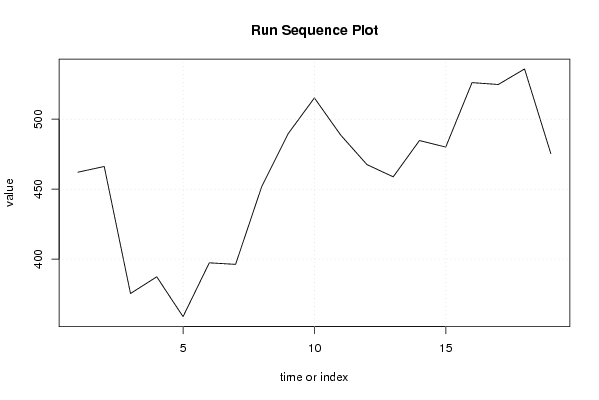PNG link Postscript link PDF link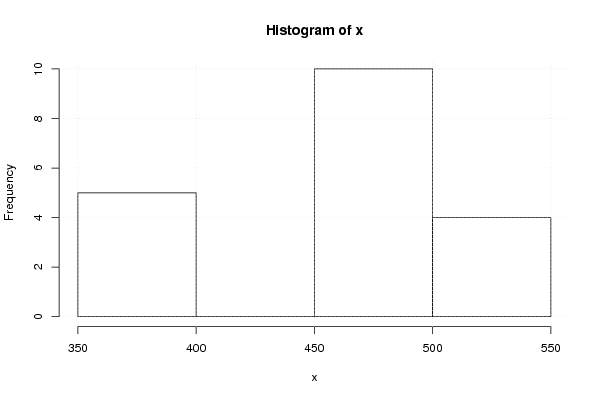PNG link Postscript link PDF link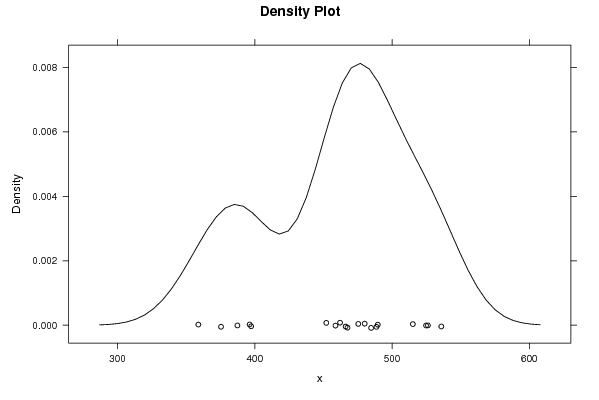PNG link Postscript link PDF link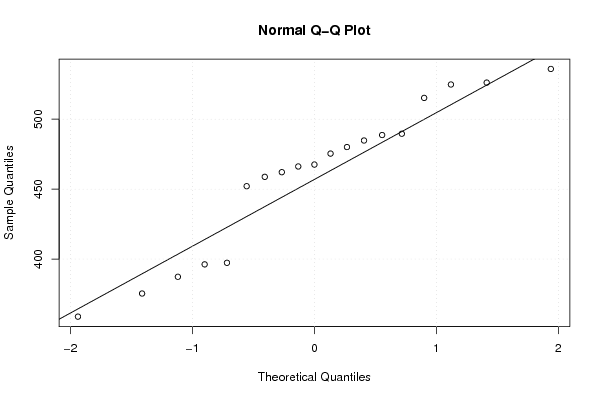PNG link Postscript link PDF link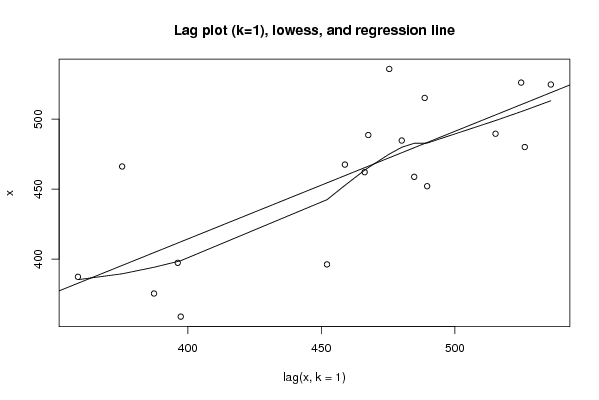PNG link Postscript link PDF link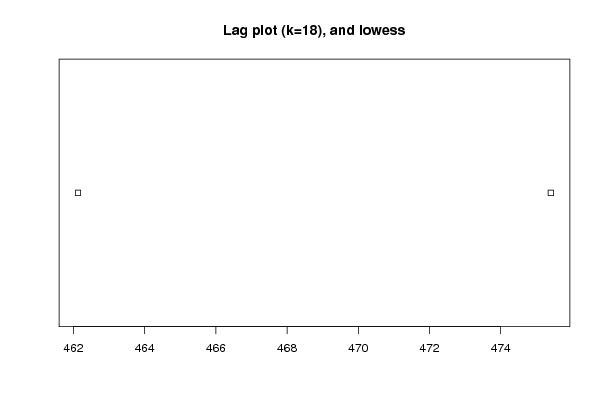PNG link Postscript link PDF link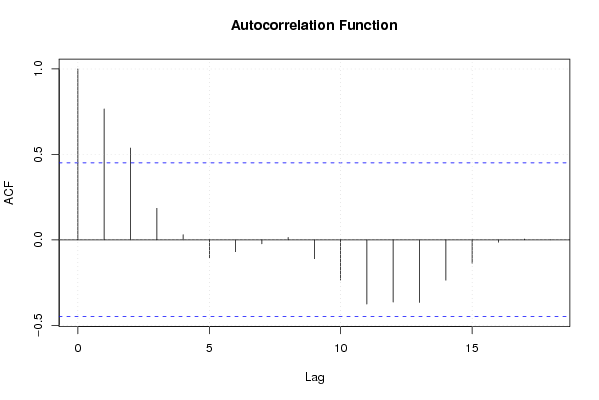PNG link Postscript link PDF link

Parameters (Session):
par1 = 0 ; par2 = 18 ;
Parameters (R input):
par1 = 0 ; par2 = 18 ;
R code (references can be found in the software module):
par1 <- as.numeric(par1)par2 <- as.numeric(par2)x <- as.ts(x)library(lattice)bitmap(file='pic1.png')plot(x,type='l',main='Run Sequence Plot',xlab='time or index',ylab='value')grid()dev.off()bitmap(file='pic2.png')hist(x)grid()dev.off()bitmap(file='pic3.png')if (par1 > 0){densityplot(~x,col='black',main=paste('Density Plot   bw = ',par1),bw=par1)} else {densityplot(~x,col='black',main='Density Plot')}dev.off()bitmap(file='pic4.png')qqnorm(x)qqline(x)grid()dev.off()if (par2 > 0){bitmap(file='lagplot1.png')dum <- cbind(lag(x,k=1),x)dumdum1 <- dum[2:length(x),]dum1z <- as.data.frame(dum1)zplot(z,main='Lag plot (k=1), lowess, and regression line')lines(lowess(z))abline(lm(z))dev.off()if (par2 > 1) {bitmap(file='lagplotpar2.png')dum <- cbind(lag(x,k=par2),x)dumdum1 <- dum[(par2+1):length(x),]dum1z <- as.data.frame(dum1)zmylagtitle <- 'Lag plot (k='mylagtitle <- paste(mylagtitle,par2,sep='')mylagtitle <- paste(mylagtitle,'), and lowess',sep='')plot(z,main=mylagtitle)lines(lowess(z))dev.off()}bitmap(file='pic5.png')acf(x,lag.max=par2,main='Autocorrelation Function')grid()dev.off()}summary(x)load(file='createtable')a<-table.start()a<-table.row.start(a)a<-table.element(a,'Descriptive Statistics',2,TRUE)a<-table.row.end(a)a<-table.row.start(a)a<-table.element(a,'# observations',header=TRUE)a<-table.element(a,length(x))a<-table.row.end(a)a<-table.row.start(a)a<-table.element(a,'minimum',header=TRUE)a<-table.element(a,min(x))a<-table.row.end(a)a<-table.row.start(a)a<-table.element(a,'Q1',header=TRUE)a<-table.element(a,quantile(x,0.25))a<-table.row.end(a)a<-table.row.start(a)a<-table.element(a,'median',header=TRUE)a<-table.element(a,median(x))a<-table.row.end(a)a<-table.row.start(a)a<-table.element(a,'mean',header=TRUE)a<-table.element(a,mean(x))a<-table.row.end(a)a<-table.row.start(a)a<-table.element(a,'Q3',header=TRUE)a<-table.element(a,quantile(x,0.75))a<-table.row.end(a)a<-table.row.start(a)a<-table.element(a,'maximum',header=TRUE)a<-table.element(a,max(x))a<-table.row.end(a)a<-table.end(a)table.save(a,file='mytable.tab')